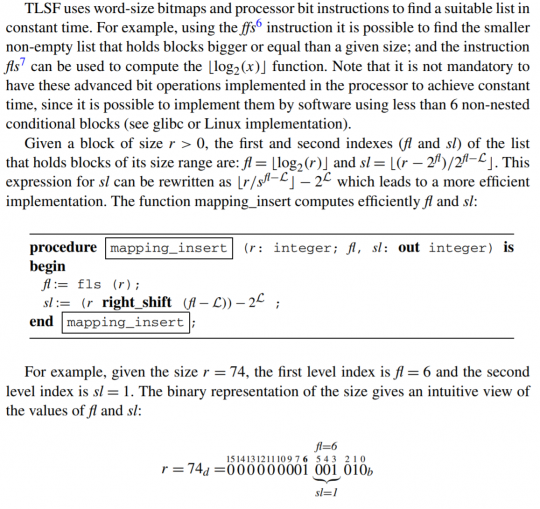# A little bit of floating point in a memory allocator — Part 1: Background

This post contains the same material as this thread of tweets, with a few minor edits.

Over my holiday break at the end of 2017, I took a look into the TLSF (Two Level Segregated Fit) memory allocator to better understand how it works. I’ve made use of this allocator and have been impressed by its real world performance, but never really done a deep dive to properly understand it.

The mapping_insert() function is a key part of the allocator implementation, and caught my eye. Here’s how that function is described in the paper A constant-time dynamic storage allocator for real-time systems:I’ll be honest: from that description, I never developed a clear picture in my mind of what that function does.

(Reading it now, it seems reasonably clear – but I can say that only after I spent quite a bit of time using other methods to develop my understanding)

Something that helped me a lot was by looking at the implementation of that function from github.com/mattconte/tlsf/.  There’s a bunch of long-named macro constants in there, and a few extra implementation details. If you collapse those it looks something like this:

```void mapping_insert(size_t size, int* fli, int* sli)
{
int fl, sl;
if (size < 256)
{
fl = 0;
sl = (int)size / 8;
}
else
{
fl = fls(size);
sl = (int)(size >> (fl - 5)) ^ 0x20;
fl -= 7;
}
*fli = fl;
*sli = sl;
}
```

It’s a pretty simple function (it really is). But I still failed to *see* the pattern of results that would be produced in my mind’s eye.

I went so far as to make a giant spreadsheet of all the intermediate values for a range of inputs, to paint myself a picture of the effect of each step :) That helped immensely.

Breaking it down…

There are two cases handled in the function: one for when size is below a certain threshold, and on for when it is larger. The first is straightforward, and accounts for a small number of possible input values. The large size case is more interesting.

The function computes two values: fl and sl, the first and second level indices for a lookup table. For the large case, fl (where fl is “first level”) is computed via fls(size) (where fls is short for “find last set” – similar names, just to keep you on your toes).

fls() returns the index of the largest bit set, counting from the least significant slbit, which is the index of the largest power of two. In the words of the paper:

“the instruction fls can be used to compute the ⌊log2(x)⌋ function”

Which is, in C-like syntax: floor(log2(x))

And there’s that “fl -= 7” at the end. That will show up again later.

For the large case, the computation of sl has a few steps:

sl = (size >> (fl – 5)) ^ 0x20;

Depending on shift down size by some amount (based on fl), and mask out the sixth bit?

(Aside: The CellBE programmer in me is flinching at that variable shift)

It took me a while (longer than I would have liked…) to realize that this
size >> (fl – 5) is shifting size to generate a number that has exactly six significant bits, at the least significant end of the register (bits 5 thru 0).

Because fl is the index of the most significant bit, after this shift, bit 5 will always be 1 – and that “^ 0x20” will unset it, leaving the result as a value between 0 and 31 (inclusive).

So here’s where floating point comes into it, and the cute thing I saw: another way to compute fl and sl is to convert size into an IEEE754 floating point number, and extract the exponent, and most significant bits of the mantissa. I’ll cover that in the next part, here.